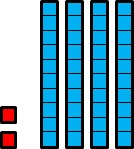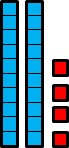# Tenths and Hundredths

Alignments to Content Standards: 5.NBT.A.1

Jossie drew a picture to represent 0.24:She said,

The little squares represent tenths and the rectangles represent hundredths, which makes sense because ten little squares makes one rectangle, and ten times ten is one hundred.
1. Explain what is wrong with Jossie's reasoning.

2. Name three numbers that Jossie's picture could represent. In each case, What does a little square represent? What does a rectangle represent?

## IM Commentary

The purpose of this task is for students to explore the relationship between tenths and hundredths (as well as the relationship between tens and hundreds). They will grapple with the common misconception that because 10 tens equals 1 hundred, then 10 tenths must equal 1 hundredth. Pushing students to link visuals with their reasoning will help them self-correct. Moreover, the teacher can assist by asking students to think about what one whole would be and reminding them that 1 tenth must represent 1 tenth of the whole and 1 hundredth must represent 1 hundredth of the whole. As students come up with representations and justifications in both parts, ask them to explain how they know their ideas make sense for tenths and hundredths.

This task also leads to a generalization. While it may be tempting to include this place value organizer from the start of the task, it will be more powerful to let students discover the patterns of our base-ten system first. If it is provided from the start, students may simply fill in following the pattern without understanding. It would be most purposeful to tie together a discussion of different student solutions with this organizer at the end and then ask the students to create a generalization about all numbers that the model in this task might represent.## Solution

1. Jossie is right that ten times ten is one hundred, but ten times one tenth is one: $$10\times 0.1 = 1$$ Since hundredths are smaller than tenths, her picture should reflect that. She could draw 2 rectangles and 4 little squares to represent 0.24.1. If the little square represents 1, then the rectangle will represent 10 and the picture will represent 42.

If the little square represents 0.1, then the rectangle will represent 1 and the picture will represent 4.2.

If the little square represents 0.01, then the rectangle will represent 0.1 and the picture will represent 0.42.